International
Tables for
Crystallography
Volume B
Reciprocal space
Edited by U. Shmueli

International Tables for Crystallography (2006). Vol. B. ch. 2.1, p. 198   | 1 | 2 |

## Section 2.1.6.3. Intensities scaled to the local average

U. Shmuelia* and A. J. C. Wilsonb

aSchool of Chemistry, Tel Aviv University, Tel Aviv 69 978, Israel, and bSt John's College, Cambridge, England
Correspondence e-mail:  ushmueli@post.tau.ac.il

#### 2.1.6.3. Intensities scaled to the local average

| top | pdf |

When the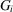's are a subset of the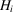's, the beta distributions of the second kind are replaced by beta distributions of the first kind, with means and variances readily found from Table 2.1.5.1. The distribution of such a ratio is chiefly of interest when Y relates to a single reflection and Z relates to a group of m intensities including Y. This corresponds to normalizing intensities to the local average. Its distribution is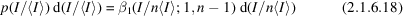in the acentric case, with an expected value of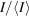of unity; there is no bias, as is obvious a priori. The variance ofis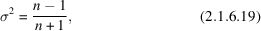which is less than the variance of the intensities normalized to an infinite' population by a fraction of the order of. Unlike the variance of the scaling factor, the variance of the normalized intensity approaches unity as n becomes large. For intensities having a centric distribution, the distribution normalized to the local average is given by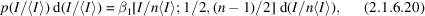with an expected value ofof unity and with variance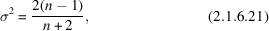less than that for an infinite' population by a fraction of about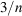.

Similar considerations apply to intensities normalized to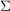in the usual way, since they are equal to those normalized to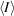multiplied by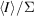.